This site uses cookies. By continuing to browse the ConceptDraw site you are agreeing to our Use of Site Cookies.
ConceptDraw Samples | Science and education — Mathematics

# Mathematics

Samples of scientific and educational mind maps, diagrams and vector illustrations on mathematics are created with ConceptDraw MINDMAP mind mapping software and ConceptDraw DIAGRAM diagramming and vector drawing software enhanced with Education solution from ConceptDraw Solution Park.

ConceptDraw DIAGRAM provides export of vector graphic multipage documents into multiple file formats: vector graphics (SVG, EMF, EPS), bitmap graphics (PNG, JPEG, GIF, BMP, TIFF), web documents (HTML, PDF), PowerPoint presentations (PPT), Adobe Flash (SWF).

## Tutorials and Solutions:

Video Tutorials: ConceptDraw Solution Park

Solutions: Mathematics for ConceptDraw DIAGRAM

## Sample 1: Flow Chart of Mathematical Algorithm — Solving Quadratic Equation

Sample of flow chart of mathematical algorithm: Solving quadratic equation.

This example is created with ConceptDraw DIAGRAM
diagramming and vector drawing software enhanced with
Mathematics solution from ConceptDraw Solution Park.

Flow charts of mathematical algorithms are used as illustrations for books, journal articles, patents, presentations and posters in mathematics and computing science, technology and education.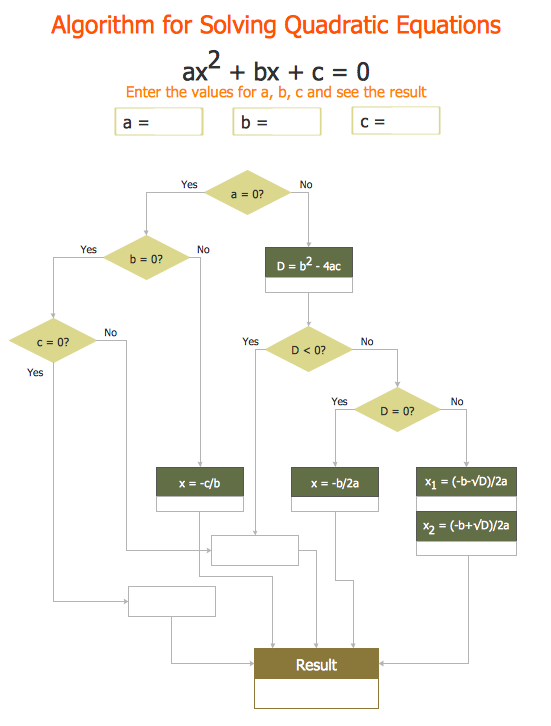## Sample 2: Mind Map — 19th Century Logic

Educational mindmap sample: 19th Century Logic.

This mathematical mind map example is created with
ConceptDraw MINDMAP mind mapping software enhanced with
Mathematics solution from ConceptDraw Solution Park.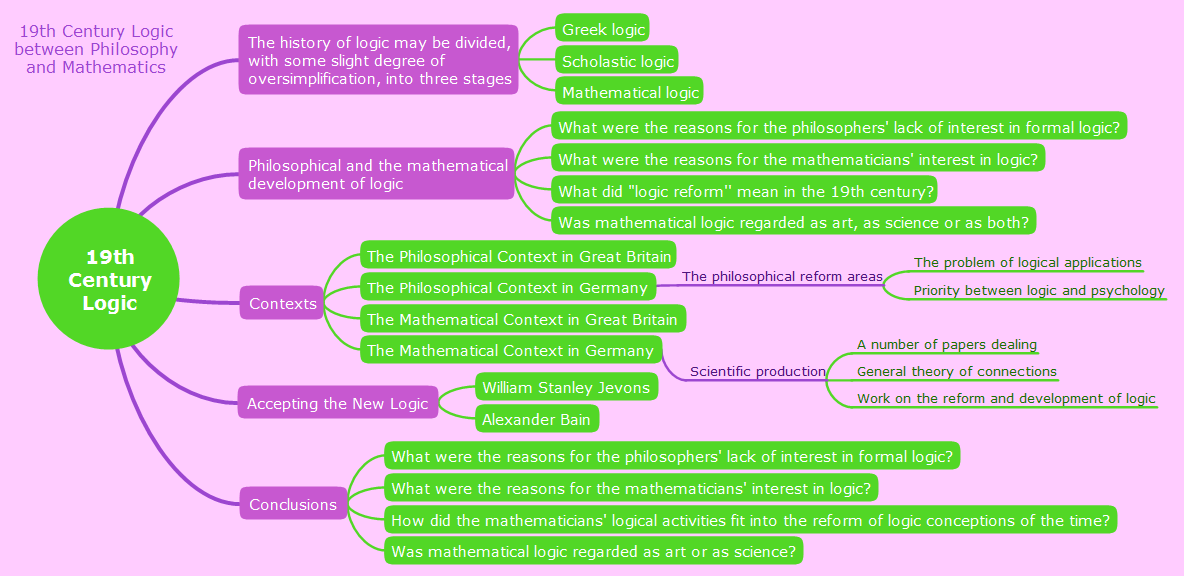## Sample 3: Mind Map — Amusements in Mathematics

Mindmap sample: Amusements in Mathematics.

This mathematical mind map example is created with
ConceptDraw MINDMAP mind mapping software enhanced with
Mathematics solution from ConceptDraw Solution Park.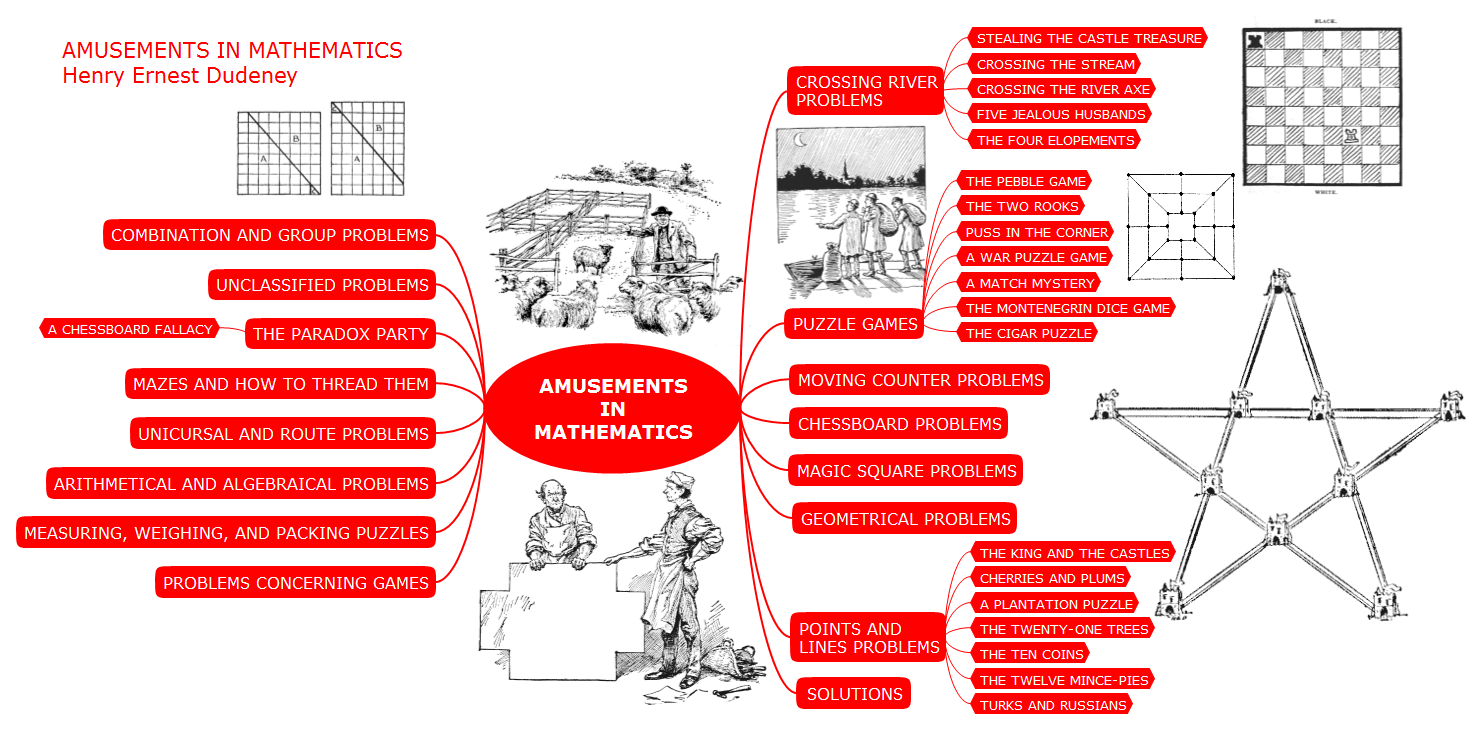## Sample 4: Mind Map — Educational Algebra

Mindmap sample: Educational Algebra.

This mathematical mind map example is created with
ConceptDraw MINDMAP mind mapping software enhanced with
Mathematics solution from ConceptDraw Solution Park.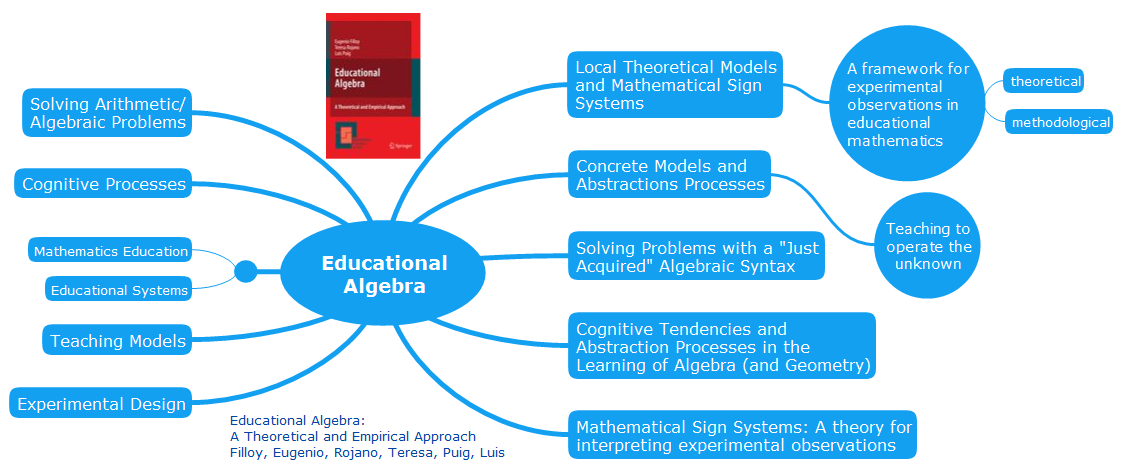## Sample 5: Mind Map — Math

Mindmap sample: Math.

This mathematical mind map example is created with
ConceptDraw MINDMAP mind mapping software enhanced with
Mathematics solution from ConceptDraw Solution Park.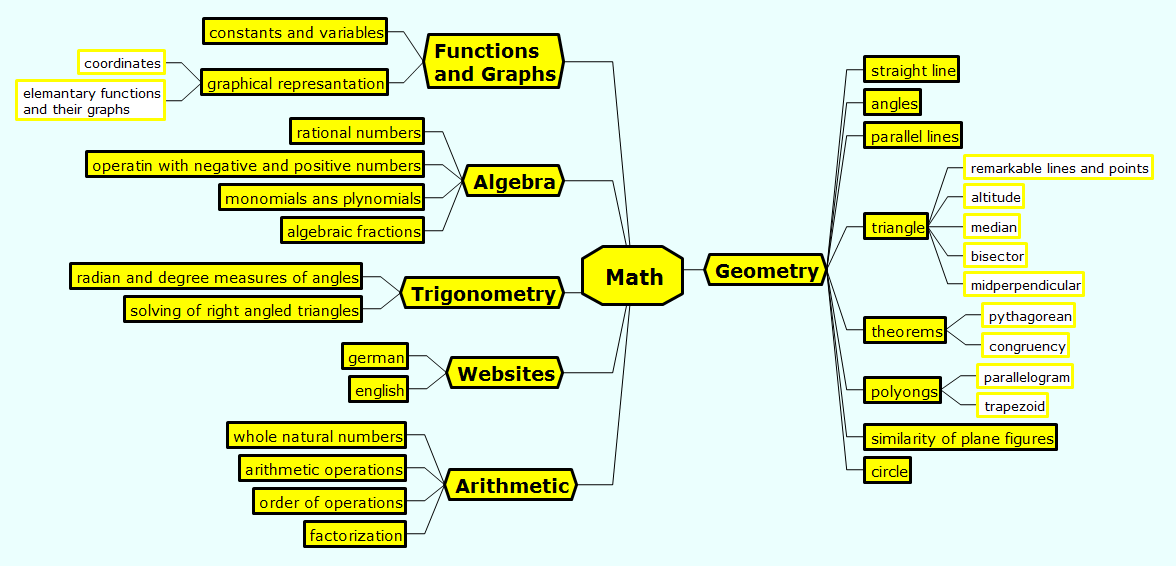## Sample 6: Mind Map — Mathematics

Mindmap sample: Mathematics.

This mathematical mind map example is created with
ConceptDraw MINDMAP mind mapping software enhanced with
Mathematics solution from ConceptDraw Solution Park.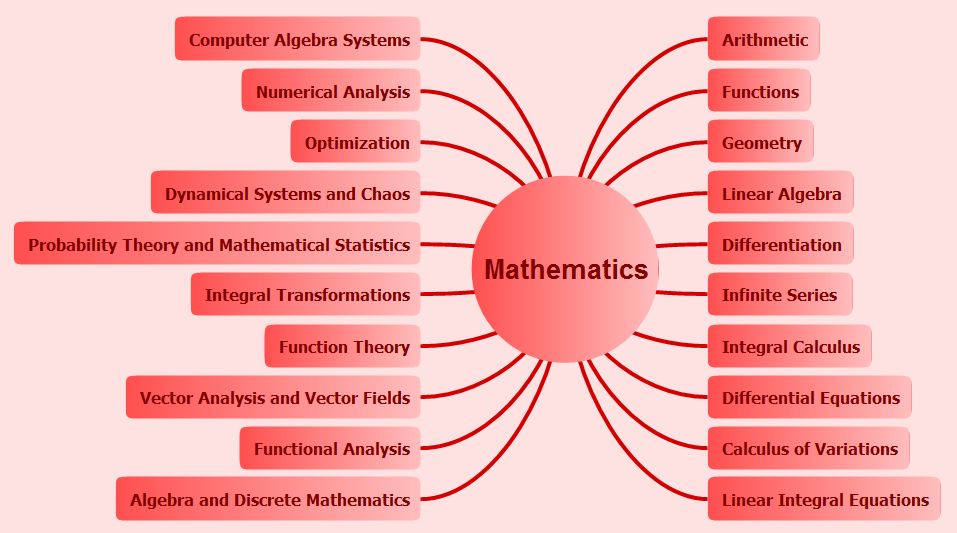## Sample 7: Mind Map — Philosophical Dimentions in Mathematics Education

Mindmap sample: Philosophical Dimentions in Mathematics Education.

This mathematical mind map example is created with
ConceptDraw MINDMAP mind mapping software enhanced with
Mathematics solution from ConceptDraw Solution Park.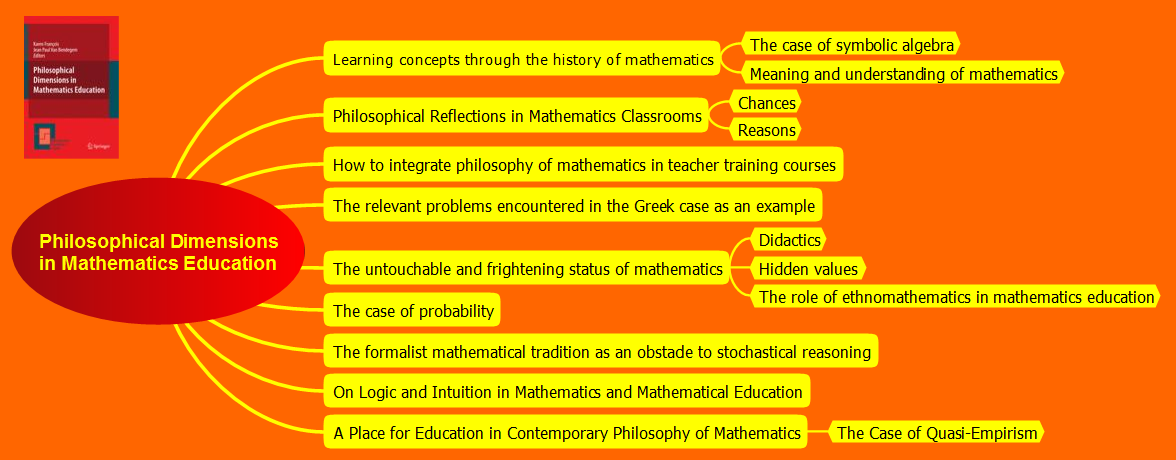## Sample 8: Mathematical Diagram — Euclid Flowchart

Mathematics sample: Euclid flowchart.

This mathematical example is created with ConceptDraw DIAGRAM diagramming and vector drawing software enhanced with Mathematics solution from ConceptDraw Solution Park.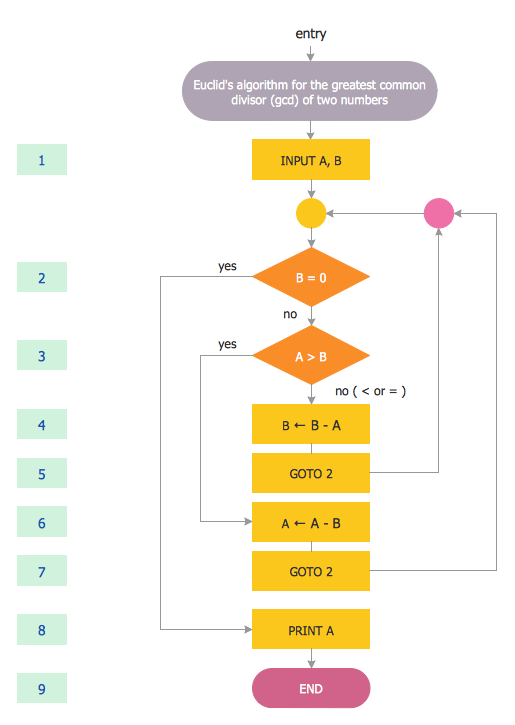## Sample 9: Mathematical Diagram — Platonic Solid

Mathematics sample: Platonic solid.

This mathematical example is created with ConceptDraw DIAGRAM diagramming and vector drawing software enhanced with Mathematics solution from ConceptDraw Solution Park.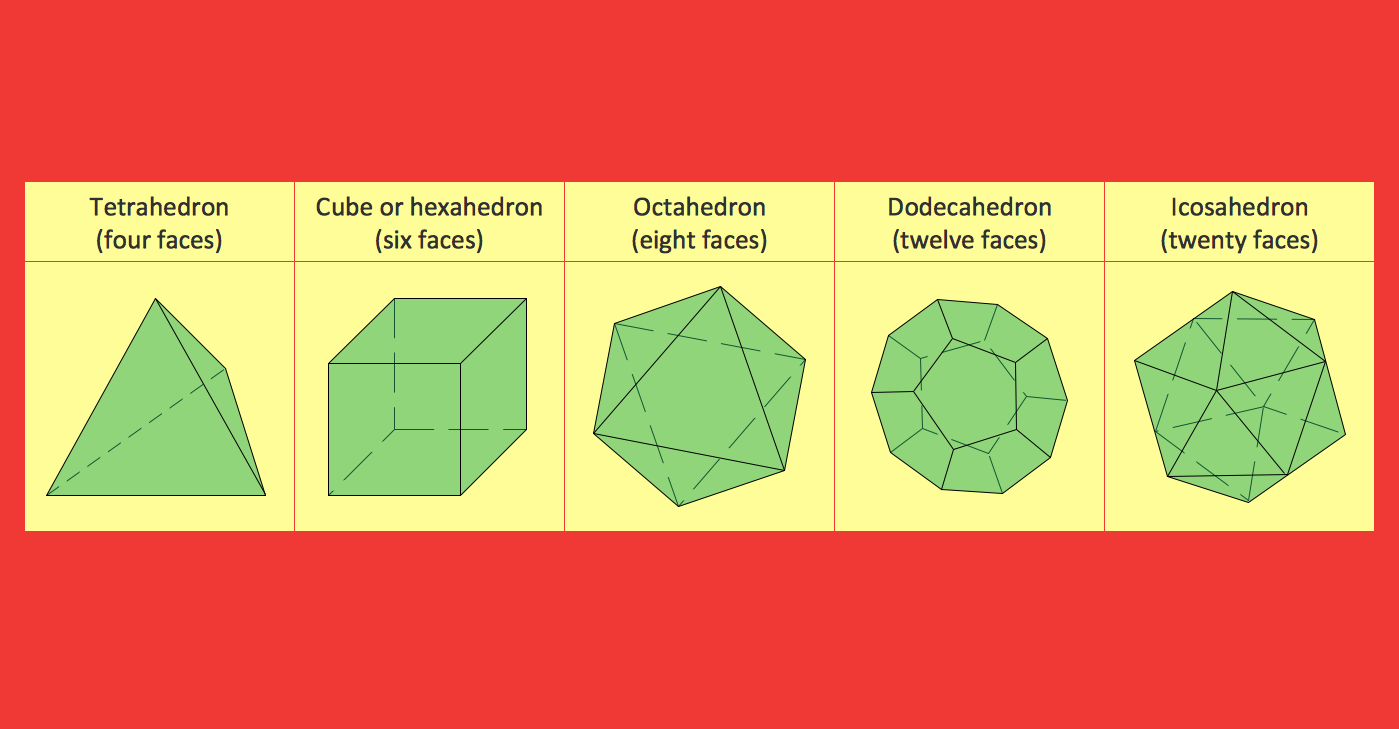## Sample 10: Mathematical Diagram — Polygon Types

Mathematics sample: Polygon types.

This mathematical example is created with ConceptDraw DIAGRAM diagramming and vector drawing software enhanced with Mathematics solution from ConceptDraw Solution Park.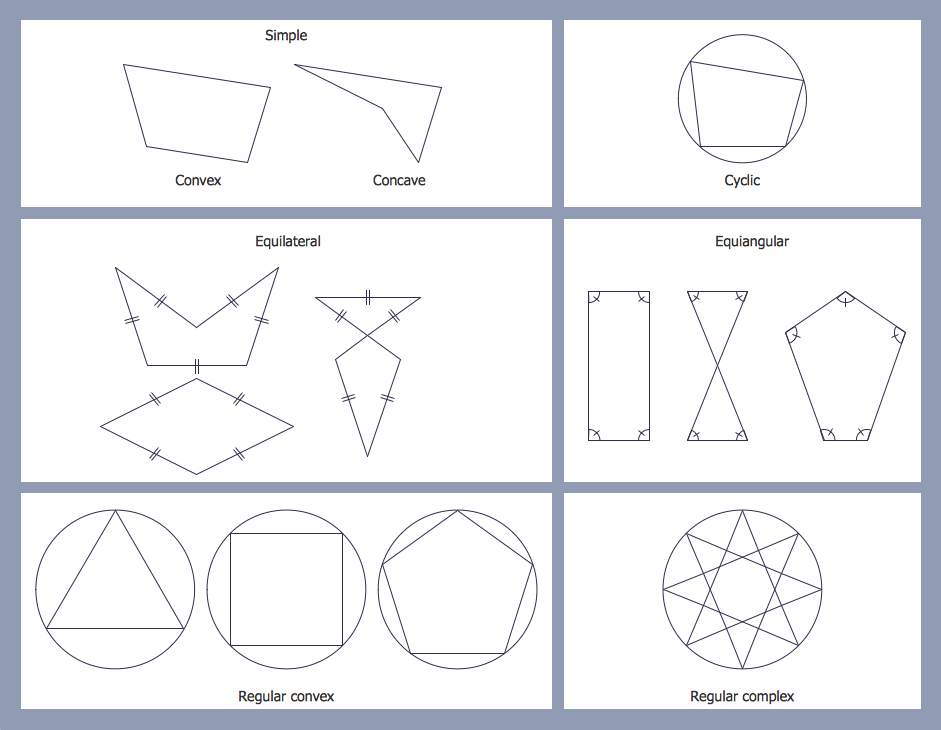## Sample 11: Mathematical Diagram — Sine Function

Mathematics sample: Sine Function.

This mathematical example is created with ConceptDraw DIAGRAM diagramming and vector drawing software enhanced with Mathematics solution from ConceptDraw Solution Park.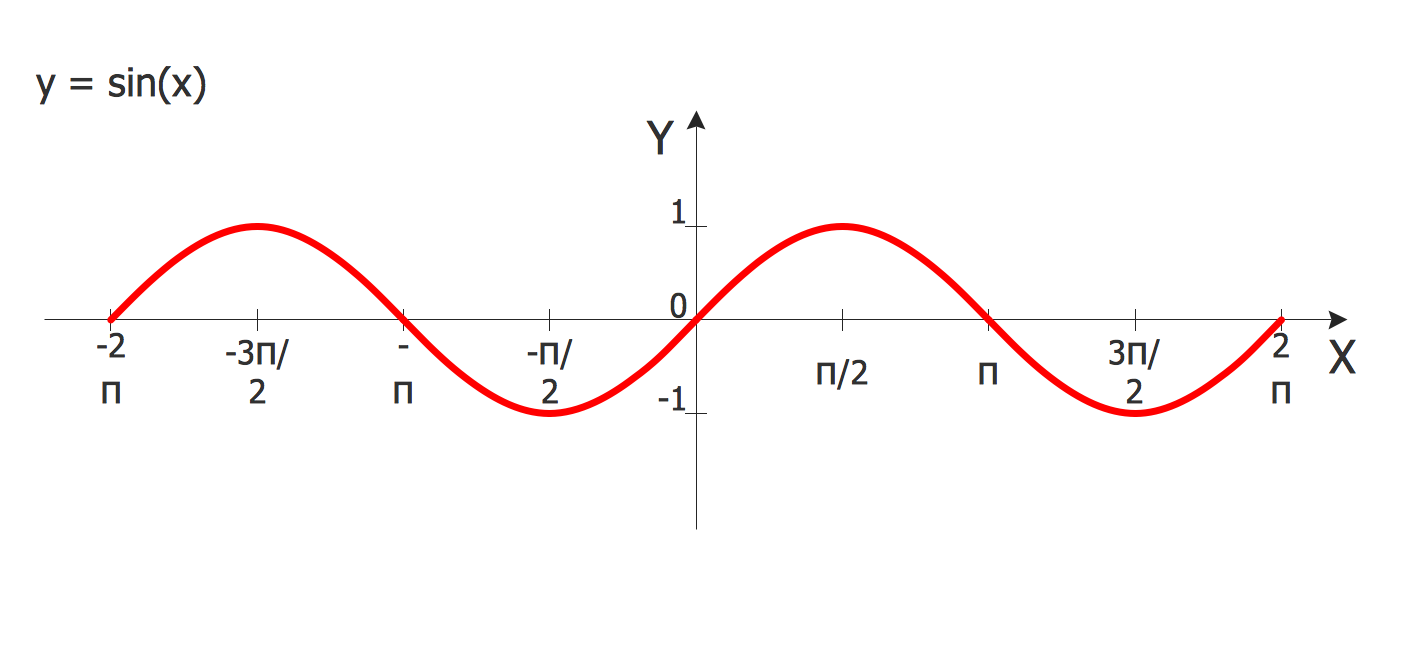All samples are copyrighted CS Odessa's.

Usage of them is covered by Creative Commons “Attribution Non-Commercial No Derivatives” License.

The text you can find at: https://creativecommons.org/licenses/by-nc-nd/3.0